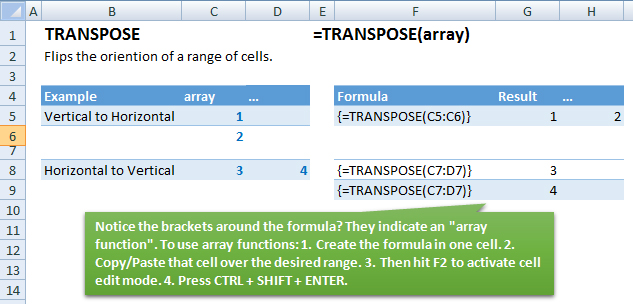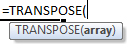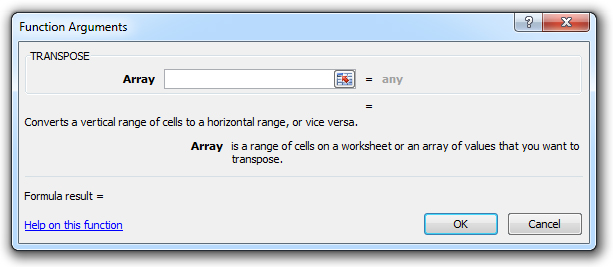### TRANSPOSE Function Examples – Excel, VBA, & Google Sheets

This Excel Tutorial demonstrates how to use the Excel TRANSPOSE Function in Excel to transpose an array, with formula examples.

## TRANSPOSE Function Description:

The TRANSPOSE Function Flips the oriention of a range of cells. Cells that were ordered left to right are now top to bottom and vis-a-versa.

## Formula Examples:Example Formula Result
Vertical to Horizontal {=TRANSPOSE(C5:C6)} 1

## Syntax and Arguments:

The Syntax for the TRANSPOSE Formula is:

`=TRANSPOSE(array)`

### Function Arguments ( Inputs ):

array – Flip the orientation of this array.

Use the TRANSPOSE Function to flip the orientation of a range of cells; Top to Bottom changes to Left to Right, or in reverse.

TRANSPOSE is a Array Formula, which requires some extra steps to use:
1. Enter The formula in the first cell.
2. Copy/Paste the formula into the other cells
3. Hit F2 (^U for MAC) to open Cell Edit Mode
4. Type CTRL + SHIFT + ENTER

If done properly, you will see brackets surrounding the array formulas:
`{=tranpose(a1:a3)}`

You can also use Paste Special: Transpose to achieve the same purpose.

## TRANSPOSE Examples in VBA

You can also use the TRANSPOSE function in VBA. Type:
`application.worksheetfunction.transpose(array)`
For the function arguments (array, etc.), you can either enter them directly into the function, or define variables to use instead.

## How to use the TRANSPOSE Function in Excel:

To use the AND Excel Worksheet Function, type the following into a cell:
`=AND(`
After entering it in the cell, notice how the AND formula inputs appear below the cell:You will need to enter these inputs into the function. The function inputs are covered in more detail in the next section. However, if you ever need more help with the function, after typing “=TRANSPOSE(” into a cell, without leaving the cell, use the shortcut CTRL + A (A for Arguments) to open the “Insert Function Dialog Box” for detailed instructions: